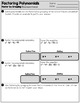# Factoring Polynomials AssessmentsSubject
Resource Type
File Type

PDF

(5 MB|18 pages)
Product Rating
Standards
Also included in:
1. This resource will help you with instruction on polynomials. Included, you will find guided notes, scavenger hunts and assessments. Below you will find a brief description of each component that is included in this bundle. For more details on each one, please click on the link provided.Guided Notes✎
\$30.50
\$24.40
Save \$6.10
• Product Description
• StandardsNEW

Use this resource to assess your students level of understanding with factoring polynomials. Below, you will find a description of each assessment. Five exit slips are also included for each type of polynomial. Detailed answer keys are provided.

x^2+ bx + c

This eight question assessment provides problems on factoring and solving x^2 + bx + c. Students are asked to factor, solve and check each solution. There is ample room to show work to support each answer. Students are asked to define the zero product property and to describe how they know their answers are correct. A problem gives students the area of a garden written as a trinomial. Students will need to determine the dimensions of the garden and the possible solutions.

ax^2+ bx + c

This eight question assessment provides problems on factoring and solving ax^2+ bx+ c. Students are asked to factor, solve and check each solution. There is ample room to show work to support each answer. Students are given a problem in which they are asked to identify the greatest common factor and to factor the simplified trinomial. A follow-up question asks students if a similar trinomial was factored correctly. The last problem asks student to determine the height of a triangle if given the area and the base. Students will draw and label a triangle, calculate the height and determine the solutions.

Perfect Square Trinomials

The perfect square trinomial questions (8 total) ask students to factor and solve problems. One question asks students to define a perfect square trinomial and to provide an example. One question asks students how many solutions exist for a perfect square trinomial.

Difference of Two Squares

Students will factor and solve difference of two squares problems. One problems asks students to explain how to identify a difference of two squares problem. Students are also asked to explain why a difference of two squares problem produces a special product. Another problem asks students why x^2+ 81 would not be considered a difference of two squares problem.

Factoring by Grouping

This four question assessment asks students to explain when it would be best to use factoring by grouping. They are asked to provide an example with their explanation. Two problems ask students to factor and solve the polynomial equation completely. They both have a difference of two squares factor within the factoring resulting in three solutions. The last question asks students to use grouping to change a^2+ 2ab + b2 to (a + b)^2.

Leaving feedback will earn you credits for future TPT resources. Follow Me to receive discounts for the first 24 hours on new resources.

Connect with me on social media!

The Clever Clover - Pinterest

The Clever Clover - Instagram

Identify zeros of polynomials when suitable factorizations are available, and use the zeros to construct a rough graph of the function defined by the polynomial.
Understand that polynomials form a system analogous to the integers, namely, they are closed under the operations of addition, subtraction, and multiplication; add, subtract, and multiply polynomials.
Total Pages
18 pages
Included
Teaching Duration
1 Week
Report this Resource to TpT
Reported resources will be reviewed by our team. Report this resource to let us know if this resource violates TpT’s content guidelines.